2017 iT 邦幫忙鐵人賽
DAY 20
1
Big Data

ggplot2散佈圖函式

geom_point(mapping = NULL, data = NULL, stat = "identity", position = "identity",
na.rm = FALSE, ...)

http://docs.ggplot2.org/0.9.3.1/geom_point.html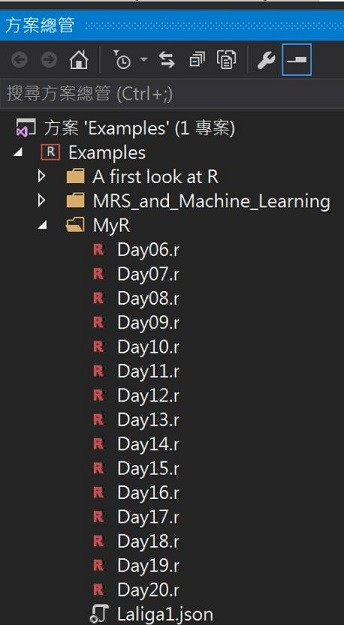散佈圖

#散佈圖(1)
ggplot(diamonds, aes(x = carat, y = price)) + geom_point()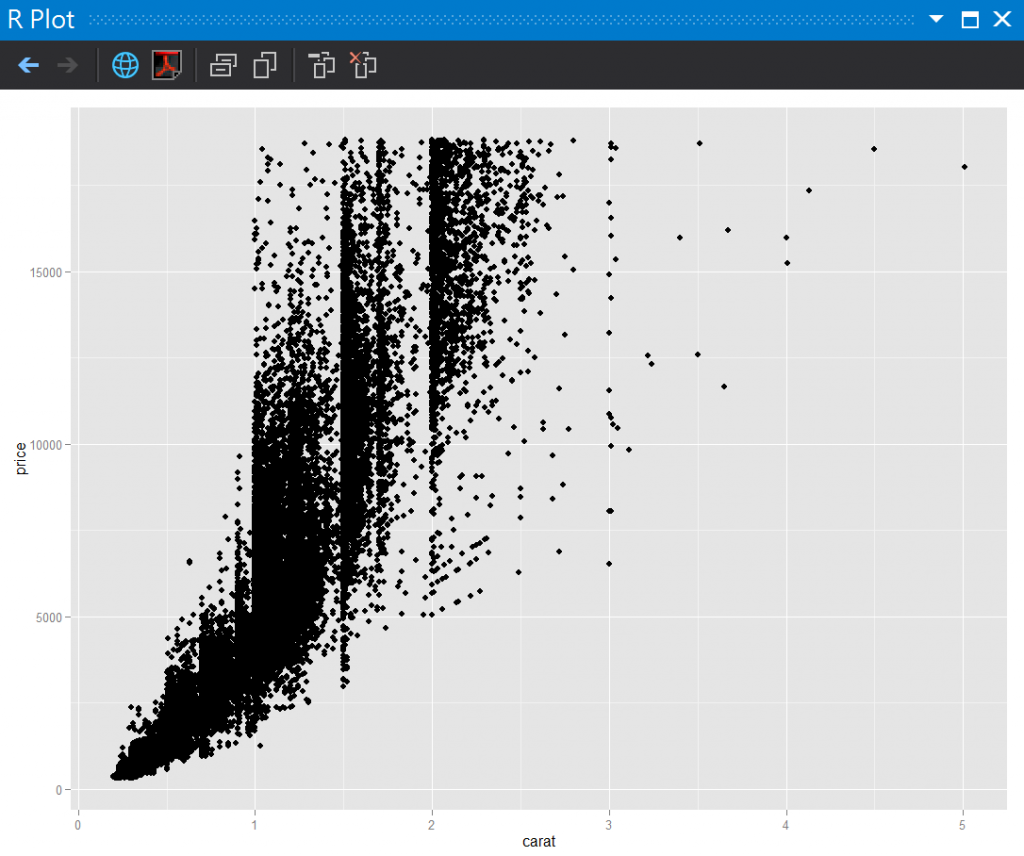密度圖

#密度圖(density)
ggplot(data = diamonds) + geom_density(aes(x = carat), fill = "#003366")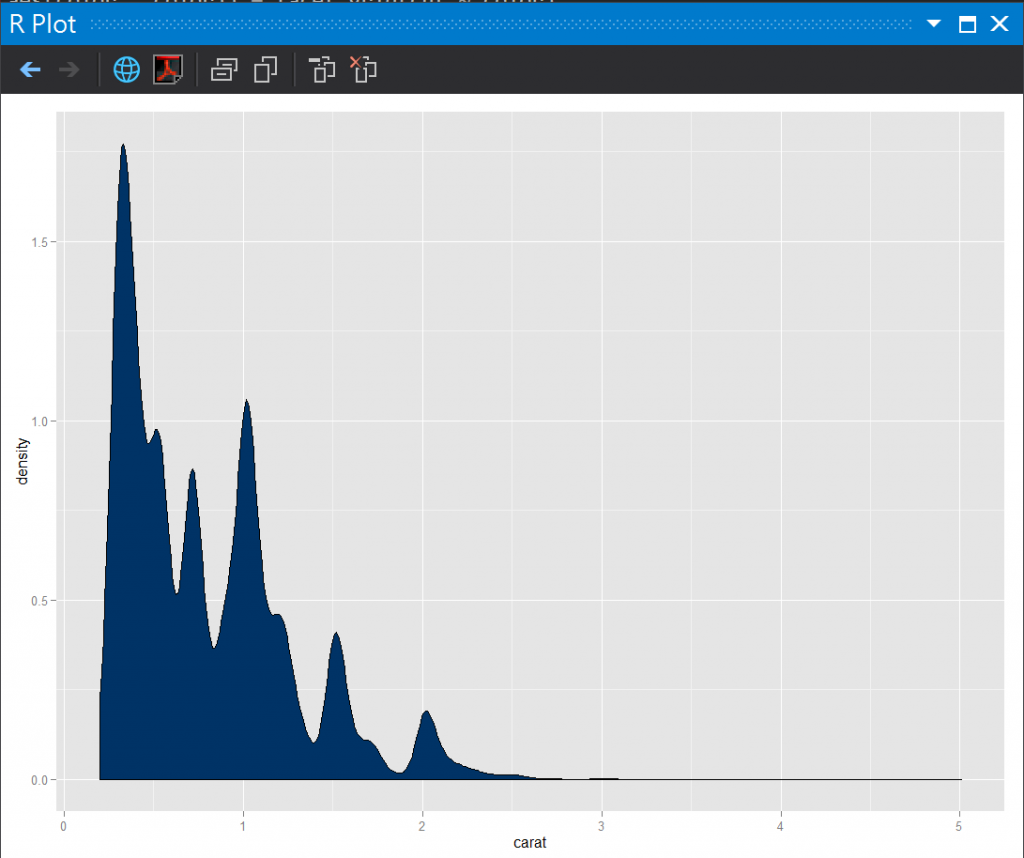多個維度

#散佈圖(2) 鑽石的淨度
ggplot(diamonds, aes(x = carat, y = price)) + geom_point(aes(color = clarity))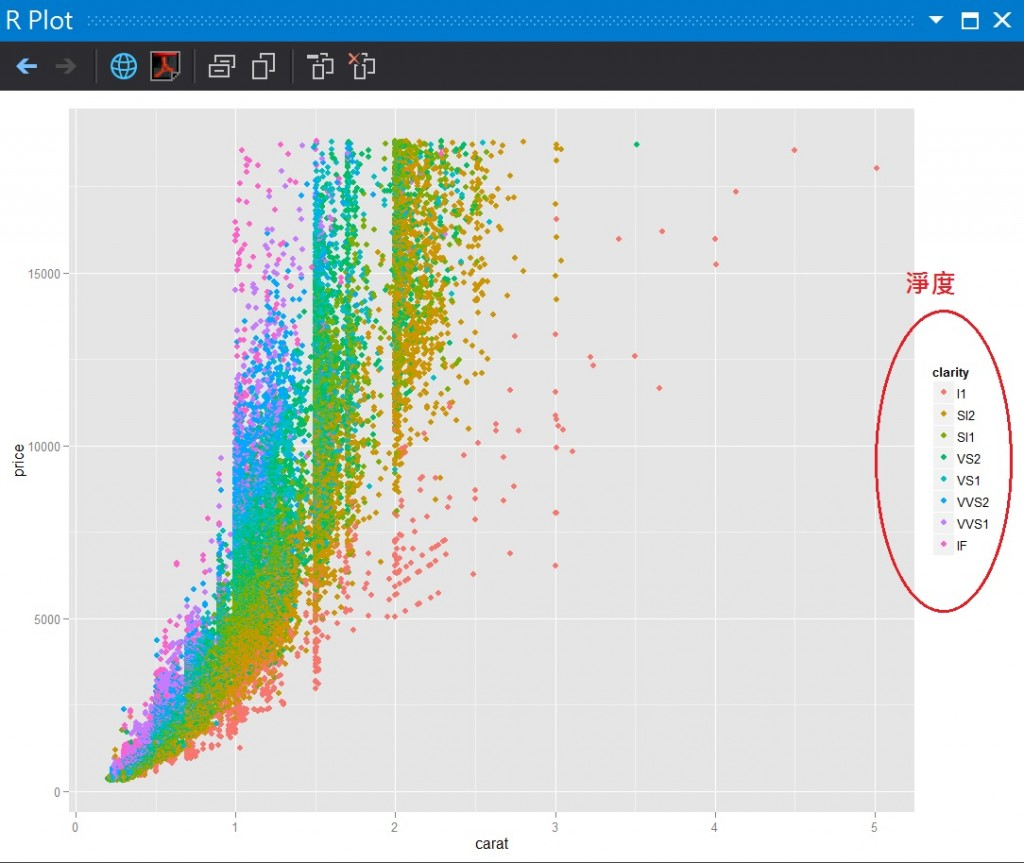ggplot(diamonds, aes(x = carat, y = price, shape = clarity)) + geom_point(aes(color = clarity))

散佈圖矩陣

#散佈圖矩陣(3) Y軸:切工  X軸:顏色
ggplot(diamonds, aes(x = carat, y = price)) + geom_point(aes(color = color)) + facet_grid(cut ~ color)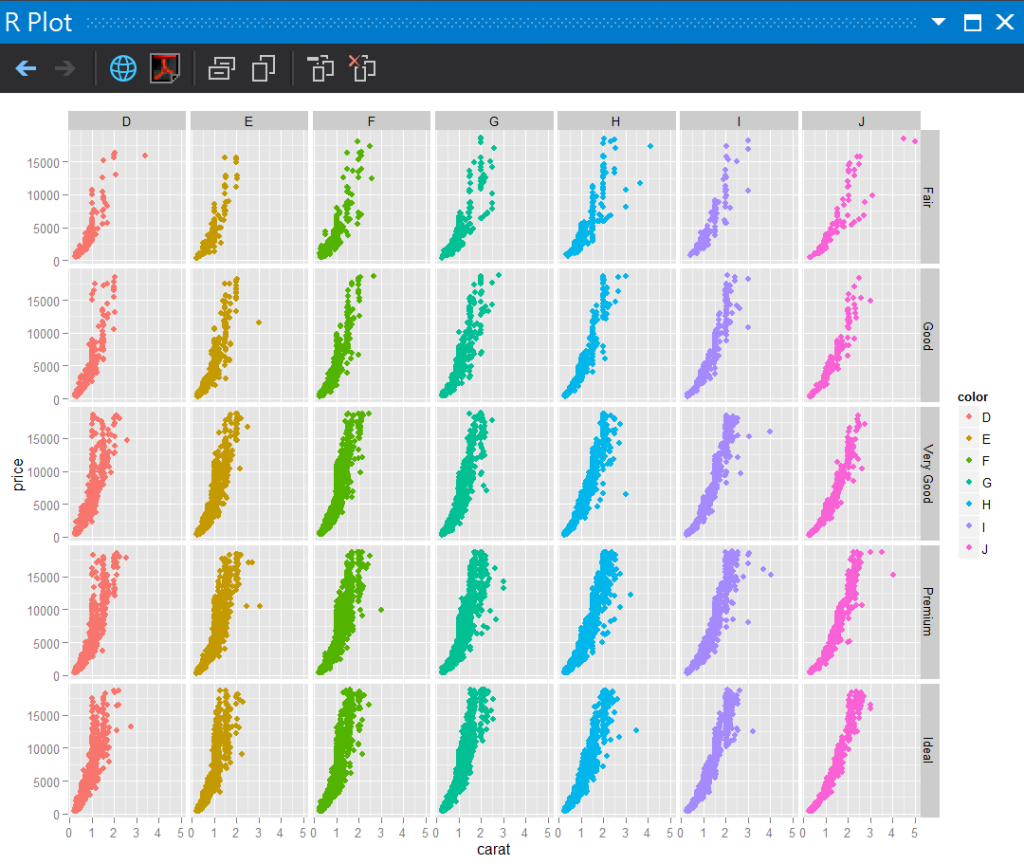散佈圖加上密度

ggplot(iris, aes(x = Petal.Length, y = Petal.Width)) + geom_point(aes(color = Species)) + stat_density2d(aes(color = Species))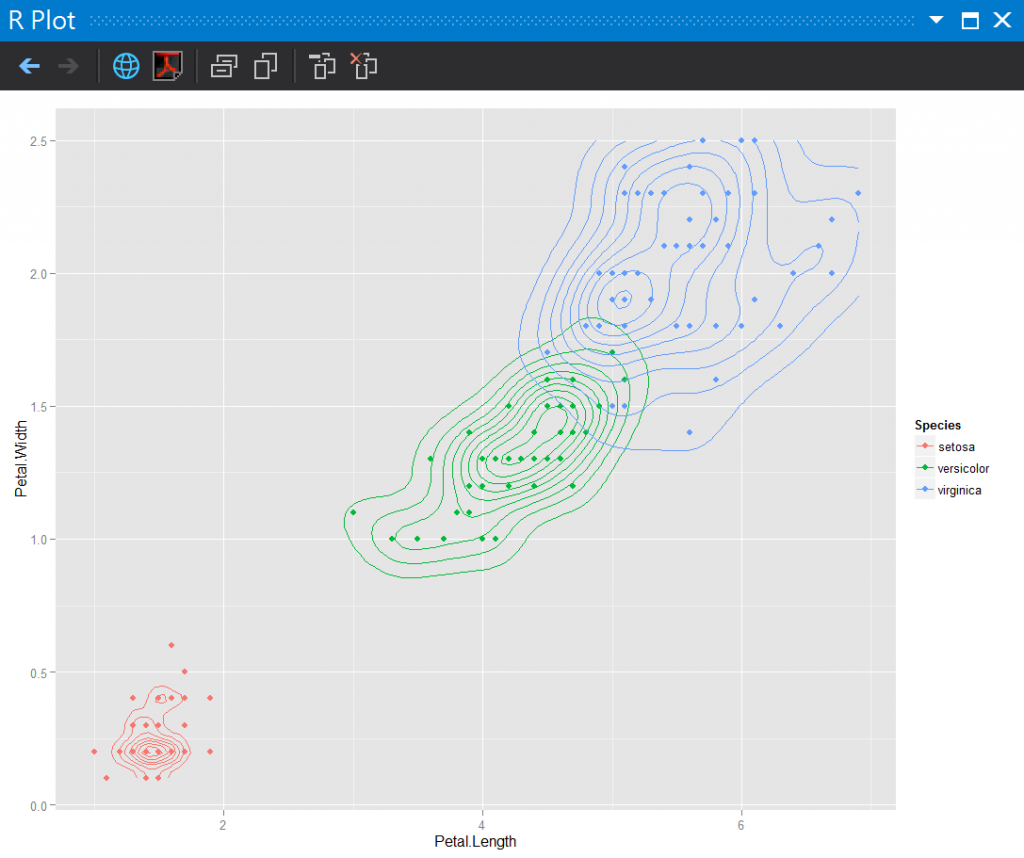點的大小

qplot(Sepal.Length, Petal.Length, data = iris, color = Species, size = Petal.Width, alpha = I(0.7))參考:

R Graphics cookbook2009.10 honeymoon攝於paris,france

R語言與機器學習見面會30

1 則留言

0